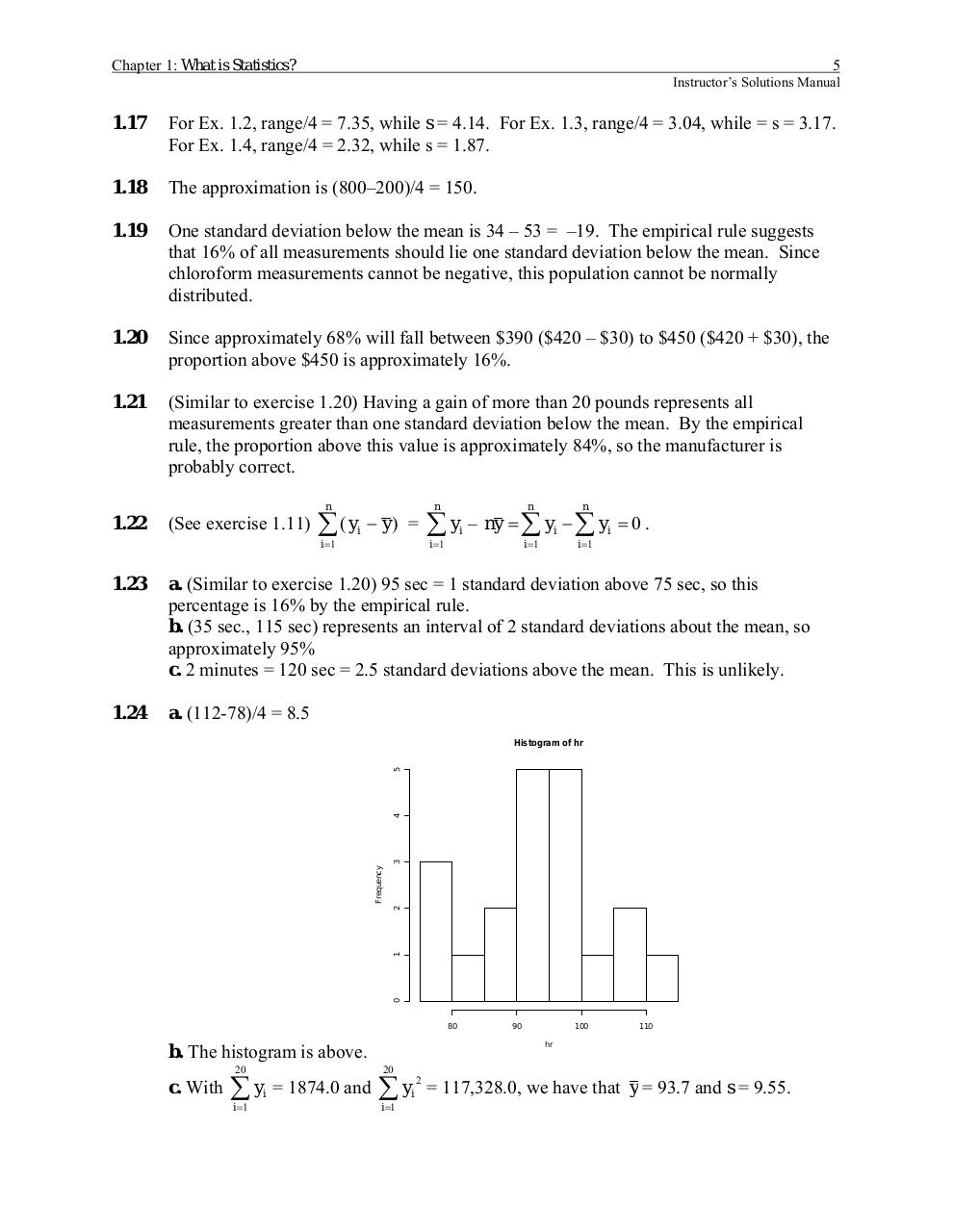Merged PDF Solutions Manual.pdfPage 1...3 4 567333

Text preview

Chapter 1: What is Statistics?

5
Instructor’s Solutions Manual

1.17

For Ex. 1.2, range/4 = 7.35, while s = 4.14. For Ex. 1.3, range/4 = 3.04, while = s = 3.17.
For Ex. 1.4, range/4 = 2.32, while s = 1.87.

1.18

The approximation is (800–200)/4 = 150.

1.19

One standard deviation below the mean is 34 – 53 = –19. The empirical rule suggests
that 16% of all measurements should lie one standard deviation below the mean. Since
chloroform measurements cannot be negative, this population cannot be normally
distributed.

1.20

Since approximately 68% will fall between \$390 (\$420 – \$30) to \$450 (\$420 + \$30), the
proportion above \$450 is approximately 16%.

1.21

(Similar to exercise 1.20) Having a gain of more than 20 pounds represents all
measurements greater than one standard deviation below the mean. By the empirical
rule, the proportion above this value is approximately 84%, so the manufacturer is
probably correct.
n

1.22

(See exercise 1.11)

∑( y
i =1

i

− y) =

n

∑y
i =1

i

n

n

i =1

i =1

– ny = ∑ yi − ∑ yi = 0 .

1.23

a. (Similar to exercise 1.20) 95 sec = 1 standard deviation above 75 sec, so this
percentage is 16% by the empirical rule.
b. (35 sec., 115 sec) represents an interval of 2 standard deviations about the mean, so
approximately 95%
c. 2 minutes = 120 sec = 2.5 standard deviations above the mean. This is unlikely.

1.24

a. (112-78)/4 = 8.5

0

1

2

Frequency

3

4

5

Histogram of hr

80

b. The histogram is above.
20

c. With

∑ yi = 1874.0 and
i =1

90

100

110

hr

20

∑y
i =1

2
i

= 117,328.0, we have that y = 93.7 and s = 9.55.